# Trigonometric Functions: Help and Review Chapter Exam

Exam Instructions:

Choose your answers to the questions and click 'Next' to see the next set of questions. You can skip questions if you would like and come back to them later with the yellow "Go To First Skipped Question" button. When you have completed the practice exam, a green submit button will appear. Click it to see your results. Good luck!

### Page 2

#### Question 6 6. What is x?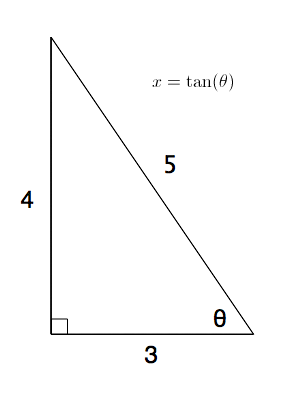#### Question 7 7. Simplify the following expression. Report the answer in degrees.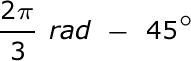#### Question 8 8. Convert the following measurement in radian to degrees.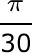#### Question 9 9. What is x?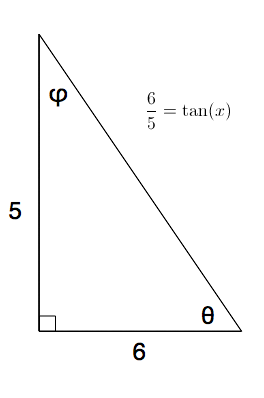#### Question 10 10. What is the following radian measure in degrees?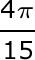### Page 3

#### Question 12 12. In the pictured triangle, side a is 9 and side b is 6. If ∠C is 26 degrees, then approximately how long is side c? Round the value of the cosine to 2 decimal places.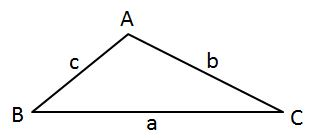#### Question 13 13. In the pictured triangle, side b is 12 and side c is 16. If ∠A is 84 degrees, then approximately how long is side a? Round the value of the cosine to 2 decimal places.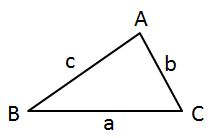### Page 4

#### Question 16 16. In the pictured triangle, ∠A is 58 degrees and ∠C is 63 degrees. If side a is 84 units long, approximately how long is side c?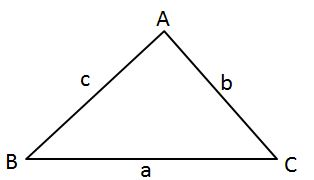#### Question 17 17. What is x?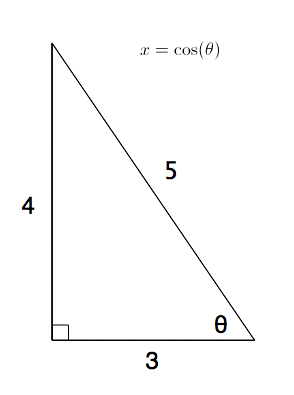#### Question 18 18. In the pictured triangle, ∠A is 137 degrees and ∠B is 28 degrees. If side b is 71 units long, approximately how long is side a?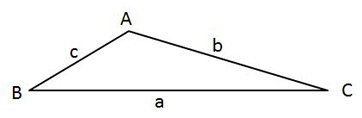#### Question 19 19. In the pictured triangle, ∠A is 81 degrees and ∠B is 67 degrees. If side a is 34 units long, approximately how long is side b?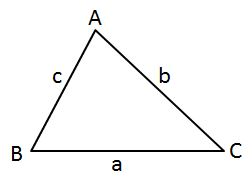### Page 5

#### Question 21 21. In the pictured triangle, ∠A is 62 degrees and ∠C is 86 degrees. If side a is 112 units long, approximately how long is side c?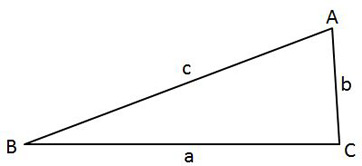#### Question 23 23. In the pictured triangle, side a is 17 and side c is 20. If ∠B is 19 degrees, then approximately how long is side b? Round the value of the cosine to 2 decimal places.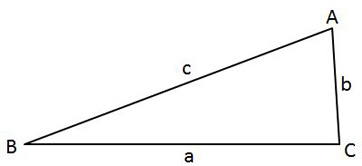### Page 6

#### Question 28 28. In the pictured triangle, side a is 32, side b is 24, and side c is 37. What is the approximate measure of ∠C? When calculating the inverse cosine, round the initial value to 2 decimal places.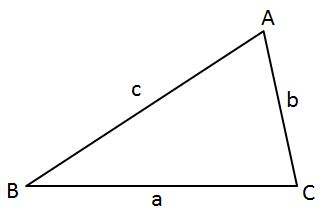#### Question 29 29. In the pictured triangle, side a is 23, side b is 18, and side c is 16. What is the approximate measure of ∠C? When calculating the inverse cosine, round the initial value to 2 decimal places.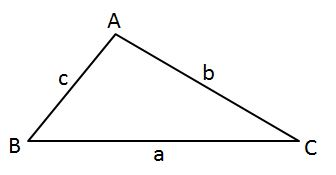#### Question 30 30. Perform the following calculation. Report the answer in radians.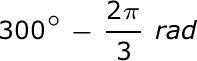#### Trigonometric Functions: Help and Review Chapter Exam Instructions

Choose your answers to the questions and click 'Next' to see the next set of questions. You can skip questions if you would like and come back to them later with the yellow "Go To First Skipped Question" button. When you have completed the practice exam, a green submit button will appear. Click it to see your results. Good luck!

Support